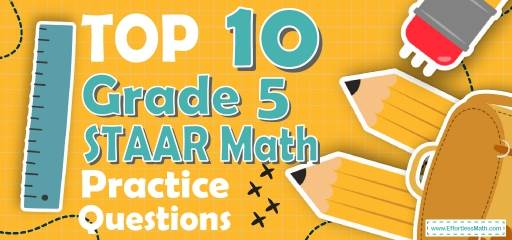# Top 10 5th Grade STAAR Math Practice QuestionsLooking for sample 5th Grade STAAR Math questions? Preparing 5th-grade students for the STAAR Math test? The best way to prepare your students for the 5th Grade STAAR Math test is to work through as many STAAR Math practice questions as possible. Here are the top 10 5th Grade STAAR Math practice questions to help students review the most important 5th Grade STAAR Math concepts. These 5th GradeSTAAR Math practice questions are designed to cover mathematics concepts and topics that are found on the actual test. The questions have been fully updated to reflect the latest 2022 5th Grade STAAR guidelines. Answers and full explanations are provided at the end of the post.

## 5th Grade STAAR Math Practice Questions

1- In a bag, there are 65 cards. Of these cards, 13 cards are white. What fraction of the cards are white?

A. $$\frac{1}{5}$$

B. $$\frac{2}{7}$$

C. $$\frac{13}{52}$$

D. $$\frac{1}{4}$$

2- Which expression is equal to $$\frac{3}{11}$$ ?

A. $$3 – 11$$

B. $$3 ÷ 11$$

C. $$3 × 11$$

D. $$\frac{11}{3}$$

3- If $$A = 20$$, then which of the following equations are correct?

A. $$A + 20 = 40$$

B. $$A ÷ 20 = 40$$

C. $$20 × A = 40$$

D. $$A – 20 = 40$$

4- How long does a 425 miles trip take moving at 50 miles per hour (mph)?

A. 4 hours

B. 6 hours and 24 minutes

C. 8 hours and 24 minutes

D. 8 hours and 30 minutes

5- 50 is What percent of 40?

A. $$20 \%$$

B. $$25 \%$$

C. $$125 \%$$

D. $$150 \%$$

6- The perimeter of the trapezoid below is 52. What is its area? _________

7- The area of a circle is $$64π$$. What is the circumference of the circle?

A. $$8 π$$

B. $$16 π$$

C. $$32 π$$

D. $$64 π$$

8- A rope weighs 800 grams per meter of length. What is the weight in kilograms of 12.2 meters of this rope? (1 kilograms = 1000 grams)

A. 0.0976

B. 0.976

C. 9.76

D. 9760

9- Solve.
$$\frac{1}{2} + \frac{4}{5} – \frac{3}{10} = ?$$

A. $$\frac{9}{10}$$

B. $$\frac{2}{10}$$

C. 1

D. 2

10- How many $$\frac{1}{4}$$ cup servings are in a package of cheese that contains $$6 \frac{1}{2}$$ cups altogether?_________

## Best 5th Grade STAAR Math Prep Resource for 2022

1- A
There are $$65$$ cards in the bag and $$13$$ of them are white. Then, $$13$$ out of $$65$$ cards are white. You can write this as: $$\frac{13}{65}$$.
To simplify this fraction, divide both numerator and denominator by $$13$$. Then:
$$\frac{13}{65}=\frac{1}{5}$$

2- B
$$\frac{3}{11}$$ means $$3$$ is divided by $$11$$. The fraction line simply means division or $$÷$$. Therefore, we can write $$\frac{3}{11}$$ as $$3 ÷ 11$$.

3- A
Plugin $$20$$ for A in the equations. Only option A works.
$$A+20=40$$
$$20+20=40$$

4- D
$$50$$ miles : $$1$$ hour
$$425$$ miles : $$425 ÷ 50 = 8.5$$ hours

5- C
Use percent formula:
$$part =\frac{percent}{100}× whole$$
$$50=\frac{percent}{100}× 40⇒ 50 = \frac{percent ×40}{100}⇒ 50 = \frac{percent ×4}{10}$$
multiply both sides by $$10$$.
$$500 =$$ percent $$×4$$, divide both sides by $$4$$.
$$125 =$$ percent

6- 104
First, find the missing side of the trapezoid. The perimeter of the trapezoid below is $$52$$. Therefore, the missing side of the trapezoid (its height) is: $$52-12-18-14=52-44=8$$
Area of a trapezoid: $$A = \frac{1}{2} h (b_{1} + b_{2}) = \frac{1}{2} (8) (12 + 14) = 104$$

7- B
Use the area and circumference of a circle formula.
Area of a circle = $$πr^2 ⇒ 64π = πr^2 ⇒ r= 8$$
Circumference of a circle = $$2πr ⇒ C = 2×8×π ⇒ C =16π$$

8- C
$$1$$ meter of the rope $$= 800$$ grams $$12.2$$ meter of the rope $$=$$ $$12.2 × 800 =9,760$$ grams $$= 9.76$$ kilograms

9- C
$$\frac{1}{2} + \frac{4}{5} – \frac{3}{10} = \frac{(5×1)+(2×4)-(1×3)}{10} =\frac{10}{10}=1$$

10- 26
To solve this problem, divide $$6\frac{1}{2}$$ by $$\frac{1}{4}$$.
$$6\frac{1}{2}÷\frac{1}{4}=\frac{13}{2}÷\frac{1}{4}=\frac{13}{2}×\frac{4}{1}=26$$

## The Best Books to Ace the 5th Grade STAAR Math Test

### What people say about "Top 10 5th Grade STAAR Math Practice Questions - Effortless Math: We Help Students Learn to LOVE Mathematics"?

No one replied yet.

X
30% OFF

Limited time only!

Save Over 30%

SAVE $5 It was$16.99 now it is \$11.99